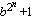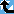# Generalized Fermat Divisors (base=6)

The Prime Pages keeps a list of the 5000 largest known primes, plus a few each of certain selected archivable forms and classes. These forms are defined in this collection's home page.

The numbers Fb,n =(with b an integer greater than one) are called the generalized Fermat numbers. (In the Prime database they are denoted GF(b,n) to avoid the use of subscripts.) It is reasonable to conjecture that for each base b, there are only finitely many such primes.

As in the case of the Fermat numbers, many have interested in the form and distribution of the divisors of these numbers. When b is even, each of their divisors must have the form

k.2m+1
with k odd and m>n. For this reason, when we find a large prime of the form k.2n+1 (with k small), we usually check to see if it divides a Fermat number. For example, Gallot's Win95 program Proth.exe has this test built in for a few choices of b.

The number k.2n+1 (k odd) will divide some generalized Fermat number for roughly 1/k of the bases b.

###Record Primes of this Type

rankprime digitswhowhencomment
111 · 210803449 + 1 3252164 L4965 May 2022 Divides GF(10803448, 6)
211 · 29381365 + 1 2824074 L4965 Mar 2020 Divides GF(9381364, 6)
329 · 27899985 + 1 2378134 L5161 Jan 2021 Divides GF(7899984, 6)
431 · 25560820 + 1 1673976 L1204 Jan 2020 Divides GF(5560819, 6)
581 · 25100331 + 1 1535355 L4965 Aug 2022 Divides GF(5100327, 6) [GG]
615 · 24246384 + 1 1278291 L3432 May 2013 Divides GF(4246381, 6)
7687 · 23955918 + 1 1190853 L5554 Feb 2023 Divides GF(3955915, 6)
8163 · 23735726 + 1 1124568 L5477 Aug 2022 Divides GF(3735725, 6)
97 · 23511774 + 1 1057151 p236 Nov 2008 Divides GF(3511773, 6)
10163 · 23250978 + 1 978645 L5161 May 2022 Divides GF(3250977, 6)
119 · 22543551 + 1 765687 L1204 Jun 2011 Divides Fermat F(2543548), GF(2543549, 3), GF(2543549, 6), GF(2543549, 12)
123 · 22478785 + 1 746190 g245 Oct 2003 Divides Fermat F(2478782), GF(2478782, 3), GF(2478776, 6), GF(2478782, 12)
131179 · 22158475 + 1 649769 L3035 Jul 2014 Divides GF(2158470, 6)
143 · 22145353 + 1 645817 g245 Feb 2003 Divides Fermat F(2145351), GF(2145351, 3), GF(2145352, 5), GF(2145348, 6), GF(2145352, 10), GF(2145351, 12)
15189 · 22115473 + 1 636824 L3784 Jan 2014 Divides GF(2115468, 6)
16107 · 22081775 + 1 626679 L3432 Dec 2013 Divides GF(2081774, 6)
17225 · 21960083 + 1 590047 L3548 Jul 2013 Divides GF(1960078, 6)
1813 · 21861732 + 1 560439 g267 Nov 2005 Divides GF(1861731, 6)
1939 · 21824871 + 1 549343 L2664 Sep 2011 Divides GF(1824867, 6)
205 · 21777515 + 1 535087 p148 Apr 2005 Divides GF(1777511, 5), GF(1777514, 6)

###References

BR98
A. Björn and H. Riesel, "Factors of generalized Fermat numbers," Math. Comp., 67 (1998) 441--446.  MR 98e:11008 (Abstract available)
DK95
H. Dubner and W. Keller, "Factors of generalized Fermat numbers," Math. Comp., 64 (1995) 397--405.  MR 95c:11010
RB94
H. Riesel and A. Börn, Generalized Fermat numbers.  In "Mathematics of Computation 1943-1993: A Half-Century of Computational Mathematics," W. Gautschi editor, Proc. Symp. Appl. Math. Vol, 48, Amer. Math. Soc., Providence, RI, 1994.  pp. 583-587, MR 95j:11006
Riesel69
H. Riesel, "Some factors of the numbers Gn = 62n + 1 and Hn = 102n + 1," Math. Comp., 23:106 (1969) 413--415.  MR 39:6813
Riesel69b
H. Riesel, "Common prime factors of the numbers An =a2n+1," BIT, 9 (1969) 264-269.  MR 41:3381